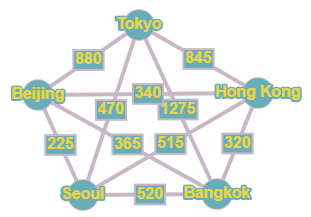# Travel Nicole wants to tour Asia. She will start and end her journey in Tokyo and visit Hong Kong, Bangkok, Seoul, and Beijing. The airfares available to her between cities are given in the table. Draw a weighted graph that represents the travel costs between cities and use the greedy algorithm to find a low-cost route.### Mathematical Excursions (MindTap C...

4th Edition
Richard N. Aufmann + 3 others
Publisher: Cengage Learning
ISBN: 9781305965584

#### Solutions

Chapter
Section### Mathematical Excursions (MindTap C...

4th Edition
Richard N. Aufmann + 3 others
Publisher: Cengage Learning
ISBN: 9781305965584
Chapter 5.2, Problem 23ES
Textbook Problem
134 views

## Travel Nicole wants to tour Asia. She will start and end her journey in Tokyo and visit Hong Kong, Bangkok, Seoul, and Beijing. The airfares available to her between cities are given in the table. Draw a weighted graph that represents the travel costs between cities and use the greedy algorithm to find a low-cost route.To determine

To represent the travel costs between the cities by a weighted graph and to find a low-cost route for the tour using the greedy algorithm.

### Explanation of Solution

Given information:

Given, Nicole wants to tour Asia.

She will start and end her journey in Tokyo and visit Hong Kong, Bangkok, Seoul, and Beijing.

The airfares available to her between cities are given in the table below:

Calculation:

Given, there are five cities out of which Nicole starts and ends at Tokyo.

Hence five vertices corresponding to the five cities are marked with respective names.

And then making the complete graph by marking every possible edge with every edge marked with the weight represented in the table between the cities.

The airfare between Tokyo and Hong Kong is \$845. Hence the edge drawn between them has a weight of 845.

Similarly marking all the edges with respective weights, the graph is given as:

To use the greedy algorithm, the graph must be Hamiltonian.

(Since, the tourist needs to visit each city only once)

A path in a Hamiltonian graph is said to be a Hamiltonian Circuit if it begins and ends at the same vertex and passes through each vertex of a graph exactly once.

The greedy algorithm states to travel along a connected edge that has the smallest weight that is not yet visited. This process continues till all the vertices are visited and returned to the starting vertex.

Here, the vertex representing Tokyo is selected.

Vertex representing Tokyo is of degree 4 and the weights of the connected edges are 845, 1275, 470, 880.

Hence, as per the Greedy Algorithm the edge with weight 470 is selected i...

### Still sussing out bartleby?

Check out a sample textbook solution.

See a sample solution

#### The Solution to Your Study Problems

Bartleby provides explanations to thousands of textbook problems written by our experts, many with advanced degrees!

Get Started

Find more solutions based on key concepts
22. If are disjoint sets, what does equal?

Mathematical Applications for the Management, Life, and Social Sciences

In Exercises 14, find the values of x that satisfy the inequality (inequalities). 4. 2x2 50

Applied Calculus for the Managerial, Life, and Social Sciences: A Brief Approach

What is a mathematical model?

Single Variable Calculus: Early Transcendentals, Volume I

Subtract the following terms as indicated. 8mn30

Mathematics For Machine Technology

The length of the curve given by x = 3t2 + 2, y = 2t3, is:

Study Guide for Stewart's Multivariable Calculus, 8th

The 2000 Census showed that the town of Grandville had a population of 1020 residents. The 2010 Census was 1170...

Study Guide for Stewart's Single Variable Calculus: Early Transcendentals, 8th

d2xdt2+dxdt+dydt=0d2ydt2+dydt4dxdt=0 x(0) = 1, x(0) = 0, y(0) = 1, y(0) = 5

A First Course in Differential Equations with Modeling Applications (MindTap Course List)

A zoo has categorized its visitors into three categories: member, school, and general. The member category ref...

Modern Business Statistics with Microsoft Office Excel (with XLSTAT Education Edition Printed Access Card) (MindTap Course List)

Alexanders Formula One interesting problem in the study of dinosaurs is to determine from their tracks how fast...

Functions and Change: A Modeling Approach to College Algebra (MindTap Course List)